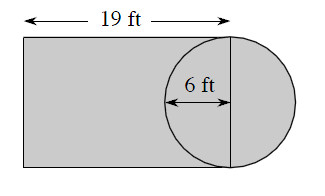### Home > MC1 > Chapter 9 > Lesson 9.3.3 > Problem9-147

9-147.

Find the area of the part of a typical basketball court shaded in the diagram below.

You can think of this as the area of a rectangle and half of a circle.

The radius of a circle is $6$ feet. This means that the diameter is $12$ feet. The diameter is also the width of the rectangle.

The area of the rectangle is $\left(19 \text{ feet}\right)\left(12 \text{ feet}\right) = 228$ square feet.

The area of the circle is $\left(6 \text{ feet}\right)^{2}π = 36$?, or approximately $113$ square feet.
We already counted half of the circle though, so we only need half of the area.
If the area of the whole circle is approximately $113$ square feet, then the area of half of the circle is approximately $56.5$ square feet.

Now we just need to add the two together: $228 \text{ square feet} + 56.5 \text{ square feet} ≈ 284.5$ square feet